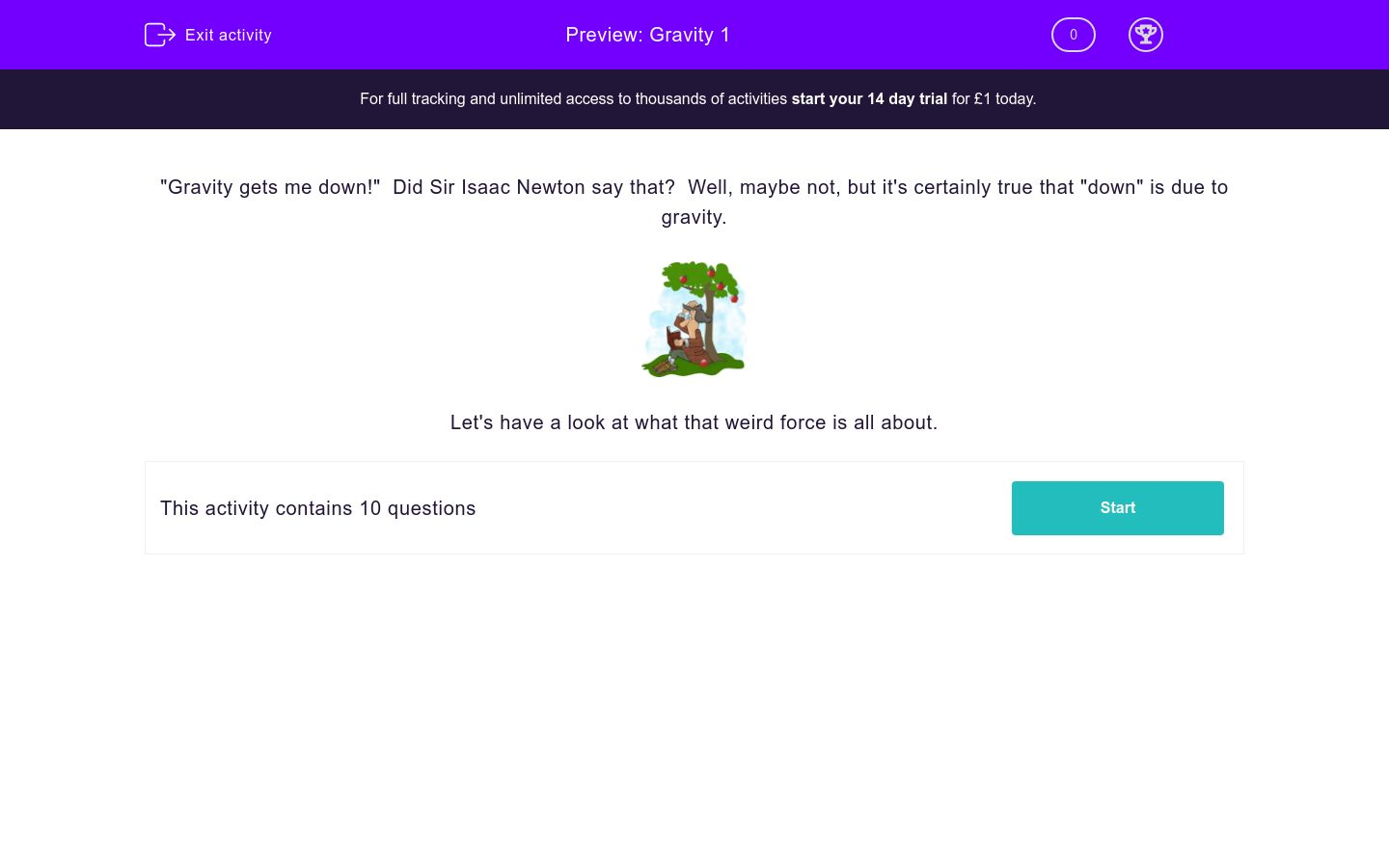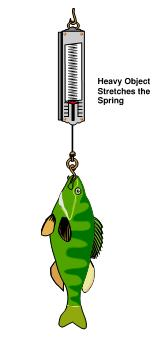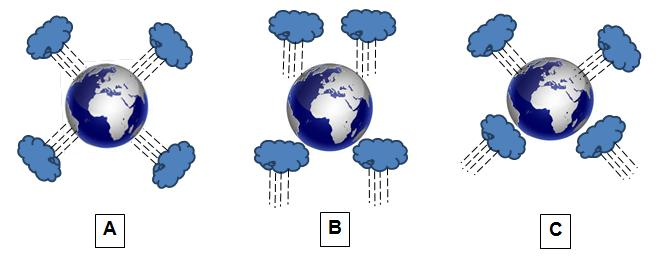# Gravity 1

In this worksheet, students will be helped to consolidate their understanding of the basics of what gravity is about: what sort of force it is, where it happens and how it is measured.Key stage:  KS 2

Curriculum topic:   Forces

Curriculum subtopic:   Gravity

Difficulty level:### QUESTION 1 of 10

"Gravity gets me down!"  Did Sir Isaac Newton say that?  Well, maybe not, but it's certainly true that "down" is due to gravity.Let's have a look at what that weird force is all about.

Gravity is an important force that attracts all objects on our planet - but where does it attract them to?

towards the centre of the Earth

towards the surface of the Earth

downwards

Without gravity where would the Atlantic Ocean go?

up in the air

up in to space

down the plug hole to the centre of the Earth

What do you think keeps a layer of air around our planet?

spinning of the Earth

weight of the air

gravity of the Earth

Which unit (or label) is used to measure the pull of gravity on an object?

Newton

kilogram

gram

Three objects were hung on a forcemeter to measure the pull of gravity on them.

Here are the results:• apple = 1N
• school bag = 60N
• fish = 10N

Which is the heaviest?

apple

school bag

fish

The same forcemeter was used to weigh three more objects. Here are the results:

• marble = 0.5N
• sugar cube = 0.25N
• tomato = 1N

Which one weighs the most?

marble

sugar cube

tomato

In the previous two questions, on which object does gravity pull the most?

school bag (60N)

fish (10N)

tomato (1N)

they're all the same

Here are three possible pictures of how rain falls on our planet:Which one do you think is right?

A

B

C

Here are some of the objects you met earlier:

• fish = 10N
• marble = 0.5N
• apple = 1N

Which one will weigh the most on the Moon?

fish

marble

appleIs the force of gravity on the Moon more, less or the same than on Earth?

more

less

the same

• Question 1

Gravity is an important force that attracts all objects on our planet - but where does it attract them to?

towards the centre of the Earth
EDDIE SAYS
Most things will drop "down" to the floor (the surface of the Earth) but what if there was a big hole in the floor - will they keep going? Of course! In Mexico there is the Cave of Swallows, the deepest vertical cave in the world and you could parachute all the way to the bottom - nearly half a kilometre! Gravity's still pulling you down there, so ... it will continue to do so to the centre of the Earth.
• Question 2

Without gravity where would the Atlantic Ocean go?

up in to space
EDDIE SAYS
Switch off gravity and everything held there by gravity disappears off into space: Big Ben, Disney World and ... the Atlantic Ocean!
• Question 3

What do you think keeps a layer of air around our planet?

gravity of the Earth
EDDIE SAYS
Without the pull exerted by gravity the air would also disappear off into space - the thin layer is held there by gravity.
• Question 4

Which unit (or label) is used to measure the pull of gravity on an object?

Newton
EDDIE SAYS
Newtons are the unit of force, named after Sir Isaac Newton who first discovered the laws of gravity, way before spaceships, back in the 1600s!
• Question 5

Three objects were hung on a forcemeter to measure the pull of gravity on them.

Here are the results:• apple = 1N
• school bag = 60N
• fish = 10N

Which is the heaviest?

school bag
EDDIE SAYS
OK, so this is an easy one! 60N is the most so that's the heaviest = school bag.
• Question 6

The same forcemeter was used to weigh three more objects. Here are the results:

• marble = 0.5N
• sugar cube = 0.25N
• tomato = 1N

Which one weighs the most?

tomato
EDDIE SAYS
Basically the tomato is 1N, the marble is half that (0.5N) and the sugar cube is one quarter of it (0.25N), so the tomato's heaviest.
• Question 7

In the previous two questions, on which object does gravity pull the most?

school bag (60N)
EDDIE SAYS
Ah, tricky! The force of gravity pulls objects to Earth at the same speed but the more massive an object is (how much 'stuff' it's made of) the greater the force of gravity on it (it needs more oomph/pull to get it moving); so the school bag contains more 'stuff' (particles, exercise books, pens, tissues, etc.!) and so has a greater pull on it.
• Question 8

Here are three possible pictures of how rain falls on our planet:Which one do you think is right?

A
EDDIE SAYS
Picture A represents reality: wherever you are on our planet, gravity pulls towards the centre of the earth, so the rain falls towards that centre (even in Australia!). Remember "down" means TOWARDS THE CENTRE OF THE EARTH.
• Question 9

Here are some of the objects you met earlier:

• fish = 10N
• marble = 0.5N
• apple = 1N

Which one will weigh the most on the Moon?

fish
EDDIE SAYS
If it's heaviest on the Earth it'll be heaviest on the Moon: that's because heavier things contain more 'stuff' (you'll learn to call it mass) and so no matter how strong the gravity they'll weigh more than lighter objects with less 'stuff' in them.
• Question 10Is the force of gravity on the Moon more, less or the same than on Earth?

less
EDDIE SAYS
It's less! Why? Well, the Moon is a tad smaller than Earth - not tiny like a weather balloon and certainly big enough to have its own gravity - but it's still pretty big - no chance of walking around it in a day or two! Its gravitational pull is about 1/6 of Earth's, so everything weighs 1/6 there of what it would weigh on Earth.
---- OR ----